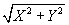Expert Modelling: Hands-on help from Rocscience Engineers. Register Here

# Displacement

The displacement options in the Interpret toolbar list, allow you to plot contours of the following displacements:

• Horizontal (X) displacement (actual values)
• Horizontal (X) displacement (absolute values)
• Vertical (Y) displacement (actual values)
• Vertical (Y) displacement (absolute values)
• Total displacement ()

Note: for Horizontal or Vertical displacements, you may plot either the absolute magnitude of the displacements, or the actual values (i.e. negative displacements will be contoured as negative values). Total displacement is always a positive value.

To view the direction of displacements, you can toggle on Deformation Vectors in the Display Options dialog. Note that, when viewing the deformation vectors:

• For Horizontal Displacement contour plots, only the X-component of the total displacement vector at each node will be displayed.
• For Vertical Displacement contour plots, only the Y-component of the total displacement vector at each node will be displayed.
• For all other contour plots (e.g. Total Displacement, Sigma1, Strength Factor, etc.) the total displacement vectors will be displayed.